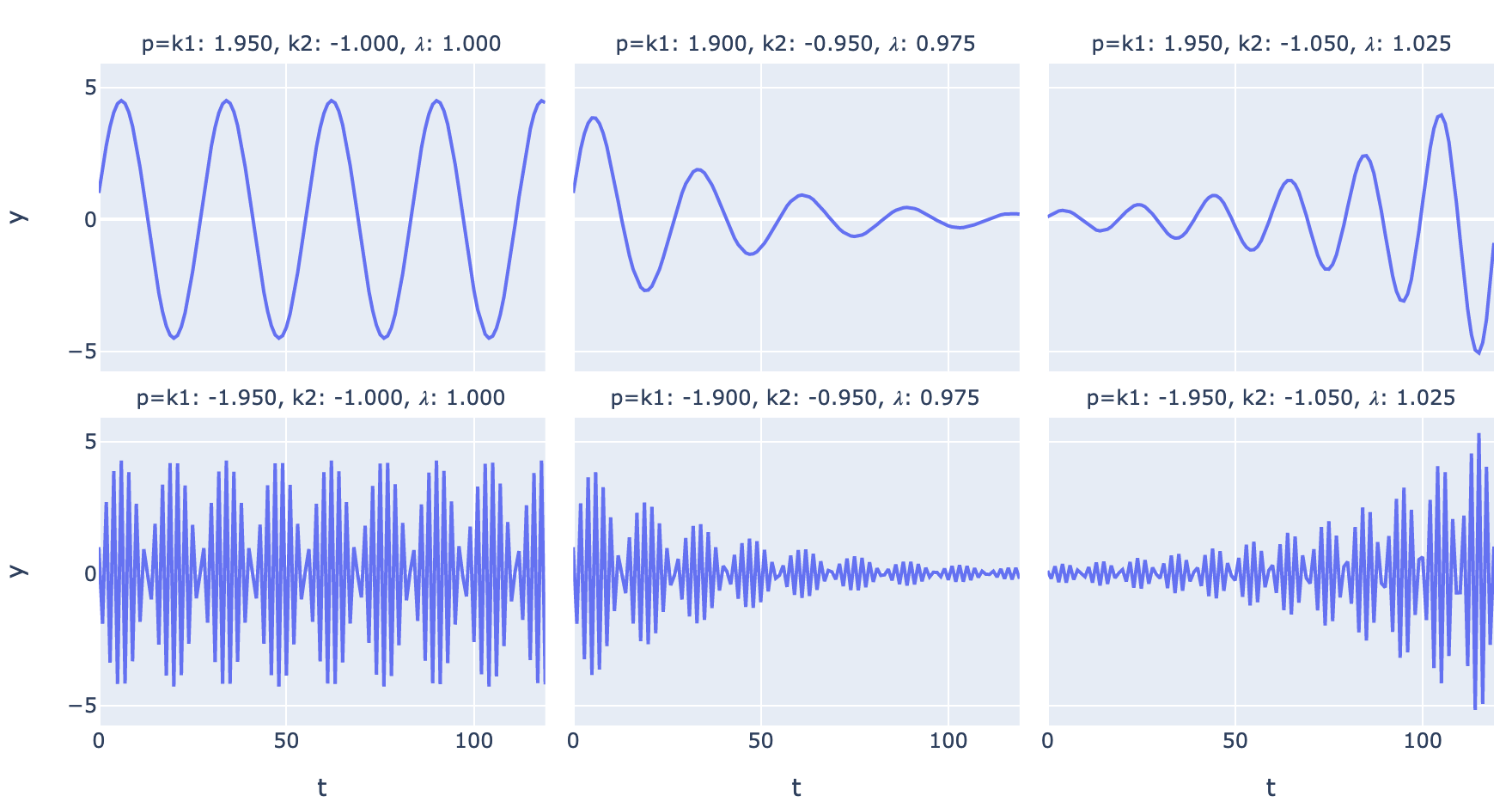• A regular time series is a function from integers to real numbers: $$y_t = f(t)$$.
• Many useful time series can be specified using linear difference equations like $$y_t = k_1y_{t-1} + k_2y_{t-2} + \dots + k_ny_{t-n}$$
• This recurrence relation has a characteristic equation (and matrix representation), whose roots (or matrix eigenvalues) can be used to write closed-form solutions like $$y_t=ax^t$$.
• Any time series combining exponential growth/decay and sinusoidal components can be modeled by a linear difference equation or its matrix representation.Fig. 1. Possible regimes for a 2nd-order linear difference equation with complex eigenvalues

So, maybe a couple of blog posts on time series analysis, starting with a little time series theory, then a practical time series analysis, and culminating in applying deep learning sequence models like transformers to time series. First, let’s refresh on the basics of time series analysis.

## 0. What is a time series?

A regular time series, where values are generated at evenly-spaced time intervals, is a function from integers to real numbers. It can be defined as a function from $$\mathbb{N} \mapsto \mathbb{R}$$ if it starts at 0, or $$\mathbb{Z} \mapsto \mathbb{R}$$ if it starts at $$-\infty$$.

Just like $$y(x)=\sin (\frac{\pi}{k} x)$$ is a real-valued function, we can have $$y(t) = \sin (\frac \pi k t)$$ where $$t \in \mathbb{N}$$.

When we build our models, we try to limit the form of our functions to something general and complex enough to fit the processes we encounter, yet simple enough that we can parameterize it to fit our data.

A simple but highly useful model is a process governed by linear difference equations. Let’s use $$y_t$$ interchangeably with $$y(t)$$.

$y_t = k_1y_{t-1} + k_2y_{t-2} + k_3 y_{t-3} + \dots k_ny_{t-n}$

where the $$k$$s are constants. Because we have $$n$$ terms like $$k_iy_{t-i}$$, this is an $$n$$th-order difference equation.

This simple construct can generate combinations of exponential trends and periodic functions, which can model many real-world processes.

## 1. First-order linear difference equations

The simplest linear difference equation is a first-order equation:

$y_t = k_1y_{t-1}$

Understanding our system and how it responds to input is not by itself sufficient to map to unique values for any $$t$$; we also need some input. The difference equation or recurrence relation models the system dynamics, which then need unique initial conditions to determine a unique time series trajectory.

For instance we can say $$k_1 = 1.1$$ and $$y_0 = 1$$. Then $$y_1=1.1$$, $$y_2 = 1.21$$ and so forth. $$y_t$$ grows by 10% at each timestep from the previous timestep, and we have exponential growth.1

If $$k_1 = 0.9$$, then $$y_1=0.9$$, $$y_2 = 0.81$$ $$y_t$$ and so forth. $$y_t$$ declines by 10% at each timestep from the previous timestep, and we have exponential decay.

We can solve a first-degree difference equation more generally. Given $$y_t = k_1y_{t-1}$$, and an initial condition $$y_0$$, let’s solve for a closed-form expression for $$y_t$$, that just depends on $$t$$ and $$k_1$$ and $$y_0$$ and doesn’t depend recursively on prior values of $$y_t$$. Based on our exploratory analysis, let’s guess that the solution is $$y_t=a \lambda^ t$$ for some $$a$$ and some $$\lambda$$, and solve for $$a$$ and $$\lambda$$.

$y_t=a \lambda ^ t$ $\iff k_1y_{t-1} = a \lambda ^ t$

Sub in the $$a \lambda^{t}$$ expression for any $$y_t$$s:

$\iff k_1 a \lambda ^ {t-1} = a \lambda ^ t$

Divide by $$a$$ to get rid of the $$a$$s:

$\iff k_1 \lambda ^ {t-1} = \lambda ^ t$ $\iff k_1 = \frac{\lambda ^ t}{\lambda ^ {t-1}} = \lambda$

That gives us the value of $$\lambda = k_1$$. We now have:

$y_t= a k_1^t$

To get the value of $$a$$, we plug in our known initial condition $$y_0$$:

$y_0 = a \lambda^ 0 = a$

So $$a = y_0$$, $$\lambda = k_1$$ and our closed-form solution is:

$y_t = y_0 k_1^t$

To repeat what we did:

We have: a difference equation relating $$y_t$$ to previous $$y_{t-j}$$s.

We want: A closed-form expression for $$y_t$$ as a function of $$t$$, without any recurrence relation.

1. Guess the form of a solution: $$y_t = a \lambda ^ t$$.

2. Solve for $$\lambda$$(s) after substituting each $$y_{t-j}$$ with $$a \lambda ^ {t-j}$$.

3. Plug in initial conditions to solve for $$a$$.

This may be a painful elaboration of the obvious. But this approach lets us solve arbitrary $$n$$th-order difference equations.

Here are plots for different values of $$k_1$$ a/k/a $$\lambda$$: